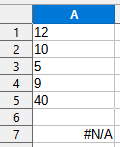# MATCH-Function should return a "index" but returns "#N/A

I try to fin out what is up and down with the MATCH-Function
I can read at LibreOffice 7.2 Help the following: " the index of the last value that is smaller or equal to the search criterion is returned. This applies even when the search array is not sorted"

Now I got this simple column (please see the attached
A1 = 12
A2 = 10
A3 = 5
A4 = 9
A5 = 40

This is the MATCH-Function: `=MATCH(9; A2:A5; 1)`

Should it not return “2” which is “the last value that is smaller or equal to the search criterion”is for first column array ascending. Click in A7 and press `Ctrl+F2` to see the Function Wizard. Why not 0, instead of 1.
Cell A1 don’t participate in this match?

Right. Therefore the first value 10 in A2 is bigger than the search value and the search stops without any match. Same with A1:A5. In spreadsheet mode we always get some match unless the search vector’s first value is smaller than the search value.

1 Like

Just try to under stand the help-file by doing
Yes it is ascending and therefore (as already wroted) it shoud return the index of the last value that is smaller or equal to the search criterion is returned

Let me ask in another wayIf i try to do “=MATCH(11 A2:A5; 1)`” then the function returns “3” This is in accordance with the help text - It searches the column array ascending and stops at the value 9 (in A4) because A5 = 40 is higher than the search criterion and returns (the relative) row number: 3

But the `=MATCH(8; A2:A5; 1)`is not behaving like that I Don’t understand why it not returns “2” cause that would also be in accordance with the help text - It searches the column array ascending and stops at the value 5 (in A3) because A4 = 9 is higher than the search criterion (8) and returns (the relative) row number: 2
But NO it returns “#N/A”

10 (A2) is greater than 8, so the search stops before it began.

1 Like

The sequence you try to find a match in is 10; 5; 9; 40

. Thisd is not ascending. At least i6t isn’t in the sense assumed here. You may convert the term to the more precise “Monotonic Ascending Step by Step From the First to the Last Element” (This does not include a “strictly”. A step is allowed to be 0. In this case the higher index is returned.) .

Of cause - silly me - forgot to skip A1 (12) - sorry to disturb

A2 was the culprit in the given example.

1 Like